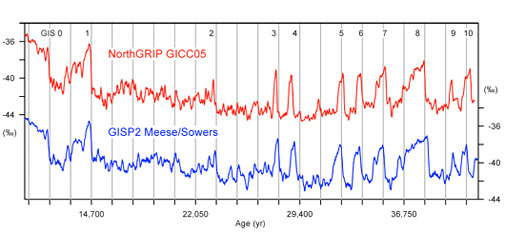# Modelling the statistics of glacial climate swings

The large climate swings during the last glacial period, the so-called Dansgaard-Oeschger (D-O) events, appear to occur with some degree of periodicity; sometimes distanced by about 1500 years, sometimes by 3000 years, and sometimes by the odd 4000 years. Many researchers claim the existence of a periodicity of 1470 years, but the result seems to depend on the statistical method used.

A group within the Centre for Ice and Climate has used a simple statistical model to test the robustness of the claimed 1470 year periodic behavior of the D-O events. By employing a statistical measure, the so-called Rayleigh's R measure, of the degree to which a time-series follows a certain beating, they found a correspondence of about 70%.

## Random jumping?

They then asked the question: How likely is this number to occur simply by chance? The answer was found by modelling the shifts as being governed by a simple Poisson process, i.e., one where waiting times are exponentially distributed with mean waiting time equal to that of the data record. This model was then used to create 1000 artificial ice cores and the R measure was calculated for each of these. The distribution of these numbers is consistent with the R measure of the real data within the high likelihood limits. This implies that there is little reason to conclude that the D-O events are periodic (or more precisely: to reject the hypothesis that they are not).The δ18O isotope records (reflecting temperatures) from NGRIP and GISP on their stratigraphic time scales. The vertical bars are separated by 1470 years and thus visually aid the evaluation of the claimed 1470 year periodicity.

## Periodic jumping?

Next, a different model of D-O event recurrence was tested – a stochastic resonance model. In this model, the system performs noise induced jumps between two states. The potential wall to be crossed for the transitions to occur changes periodically and the degree to which this periodicity stands out is determined by the amplitude of the random noise introduced. The latter was constrained by demanding that the model on average produces the correct number of events in the artificial ice core records. The distribution of R measures obtained with this model is not as compatible with the actual R measure of 70% (although they cannot be used to rule out the existence of a periodicity).

## External or internal causes of D-O events

From the above, it was concluded that a random process matches the data statistics at least as well as is one involving a periodic beating. This has important consequences for further research in D-O events: If they were periodic, they would most likely be forced by some astronomical phenomenon while randomly recurring events can be the result of internal dynamics in the climate system. Read the paper (Abstract) or contact Peter Ditlevsen.